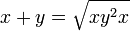# Baer correspondence establishes a correspondence between 2-powered twisted subgroups of the group and 2-powered subgroups of additive group of the Lie ring

## Statement

Consider the Baer correspondence. This is a correspondence of the form:

Baer Lie groups (defined as 2-powered groups of nilpotency class at most two)$\leftrightarrow$ Baer Lie rings (defined as 2-powered Lie rings of nilpotency class at most two)

This correspondence establishes an element-by-element bijection between the Baer Lie group and the Baer Lie ring. This bijection induces a correspondence:

2-powered twisted subgroups of the Baer Lie group$\leftrightarrow$ 2-powered subgroups of the additive group of the Baer Lie ring

## Proof

### The correspondence preserves closure under identity and inverses

Since the identity element and inverses are exactly the same for the group and the Lie ring. Hence, the correspondence preserves the property of being a symmetric subset. Explicitly:

Symmetric subsets of the Baer Lie group$\leftrightarrow$ Symmetric subsets of the Baer Lie ring

### The correspondence preserves 1-closed subsets

Powers have the same meaning on both sides. Hence, we get a correspondence:

1-closed subsets of the Baer Lie group$\leftrightarrow$ 1-closed subsets of the additiv group of the Baer Lie ring

### The correspondence preserves 2-powering for 1-closed subsets

This is immediate.

### The correspondence relates 2-powered twisted subgroups and 2-powered subgroups of the additive group

We can deduce this immediately from either of these formulas for Lie ring addition in terms of group multiplication:$x + y = x^{1/2}yx^{1/2}$$x + y = \sqrt{xy^2x}$